Mathematical Induction ExamplesMathematical Induction Examples

Learn how to use Mathematical Induction in this free math video tutorial by Mario’s Math Tutoring. We go through two examples in this video.

0:30 Explanation of the 4 Steps of Mathematical Induction
2:12 Example 1 Mathematical Induction Problem
2:35 Step 1 Show True for n=1
3:22 Step 2 Mathematical Induction Assumption
4:19 Step 3 Show True For n=k+1
7:44 Step 4 By the Process of Mathematical Induction…
8:35 Example 2
13:20 Difference between the meaning of k and n

Looking to raise your math score on the ACT and new SAT?
Check out my Huge ACT Math Video Course and my Huge SAT Math Video Course for sale at
http://mariosmathtutoring.teachable.com

http://www.mariosmathtutoring.com

* Organized List of My Video Lessons to Help You Raise Your Scores & Pass Your Class. Videos Arranged by Math Subject as well as by Chapter/Topic. (Bookmark the Link Below)
http://www.mariosmathtutoring.com/free-math-videos.html

algebra tutors,mathematical induction examples,example of mathematical induction,how to use mathematical induction,examples of mathematical induction,mathematical induction example,mathematical induction,process,mathematical proof,proof,proofs,prove,how to prove by mathematical induction,assumption,precalculus,math,maths,how to,video,free,tutorial,induction,mario’s math tutoring

algebratutors.org

34 Responses to “Mathematical Induction Examples”

1.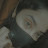Arsi Mxhi says:

thanks professor X form pakistan

2.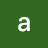arati totad says:

Thank u sir u explained why to take k and k+ 1

3.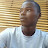Eavy Junior says:

I love this man's videos, his humble expression and detailed explanations are extra ordinary

4.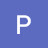Poni Murye says:

thank you so much! you really simplified it and now I understand. i can finally do my homework!

5.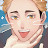esouvedot says:

Thank you so much for this!! I'm still in high school, for our Pre-Calculus and it's basically self-learning but this just made me understand it more. Thank you very much!!💞

6.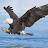Chrisogonas O. Mc'Odhiambo says:

Great and clear illustration! Thx

7.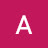Asher Crix says:

How to make a problem with your own sir??

8.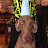Polar Panda says:

Love you man, helping me pass my pre calc test that I have next period, thnx 👌

9.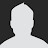Gene Eric says:

BIG LEVEL HELP PROFESSOR MARIO, GREETINGS AND THANKS FROM TUSCON ARIZONA

10.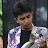Mohammad Axel says:

Thanks

11.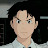dedoomx says:

My n=1 doesn't work. I mean, it goes like 5 = -5. And so on, all of em arent equal. Does that mean I don't need to proceed on step 2 because there's no sense anymore? Doesn't equal at first at all.

12.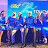Alfred Wotlolan says:

The Best explanation on Mathematical Induction! Thank you Sir!

13.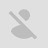manjoe lol says:

this guy makes me feel a lot better about discrete structures

14.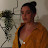xxnikixx says:

you have saved my life Mr Mario sir

15.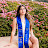Katerina Press says:

omg my tutor has been trying to teach me this but I couldn't understand until now, thank you so much!

16.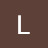Lata Shastri says:

Sir look like HITMAN,,

17.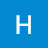Hussein Cheaito says:

I love you Mario.

18.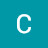Katerina S says:

Great explanation! Thank you!

19.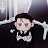DinoWarrior says:

Thanks sir for the second example

BTW ,am I the only one ,who have this topic in their class 11th syllabus 🤣🤣🤣

20.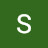Sleepy Man says:

At 6:22 I don't understand how to get from 3K(K+1) + 6(K+1) to 3(K+1)(K+2)? I can get from 3K(K+1) + 6(K+1) into 3K^2 + 9K + 6K and also I can see that 3(K+1)(K+2) is also 3K^2 + 9K + 6K, but what I do not understand is how can I get straight from 3K(K+1) + 6(K+1) to 3(K+1)(K+2)? I know it is some simple math trick, that everyone (except me) knows by heart but it would have taken like 30sec to explain it in the video.

21.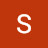Saleem Ismail kugu says:

Please sir help me solve this question. I'm having difficulties here trying to solve it.

x-12, 3x-2/7, 3x+2/7, Bx+6. What is the value of B.

22.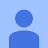Kirti Kanta says:

Thank you sir

23.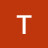Tabib Hassan says:

24.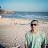Leandro Evangelista says:

thanks for this video, it's exactly what i was searching

25.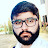Alpha Khan says:

Was it that easy ?? and i just feel shame on myself i could never understand this…… Thank you sooo much ….

26.K. B. S Srikar says:

THANK YOU SO MUCH SIR !!!!!!!

after watching so manyyyyyyyyy videos your explanation is the BEST!!!!!!!!! thank you a lot sir ,'

have a nice day!!

27.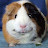One above All says:

is there is any use of M.I in real life

28.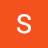Seraph.Winter says:

Is it ok if we put step 2 and step 3 as one step together?

29.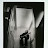geraldillo says:

You made it easy to understand, thanks!

30.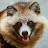TanukiSamurai says:

Was unable to perform induction for my college Discrete Math course, now I can do it with ease. Thank you 🙂

31.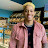Emma Chiemelie says:

Incredible

32.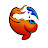cyclofish says:

can anybody explain the second example? why is the left side 2^0 instead of 1 + 2^0?

33.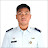Verson says:

umay.

34.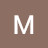Mārtiņš Mārcis says:

Couldn't understand for couple hours and then learned in 12 minutes, thats nice. Thank you soo much!